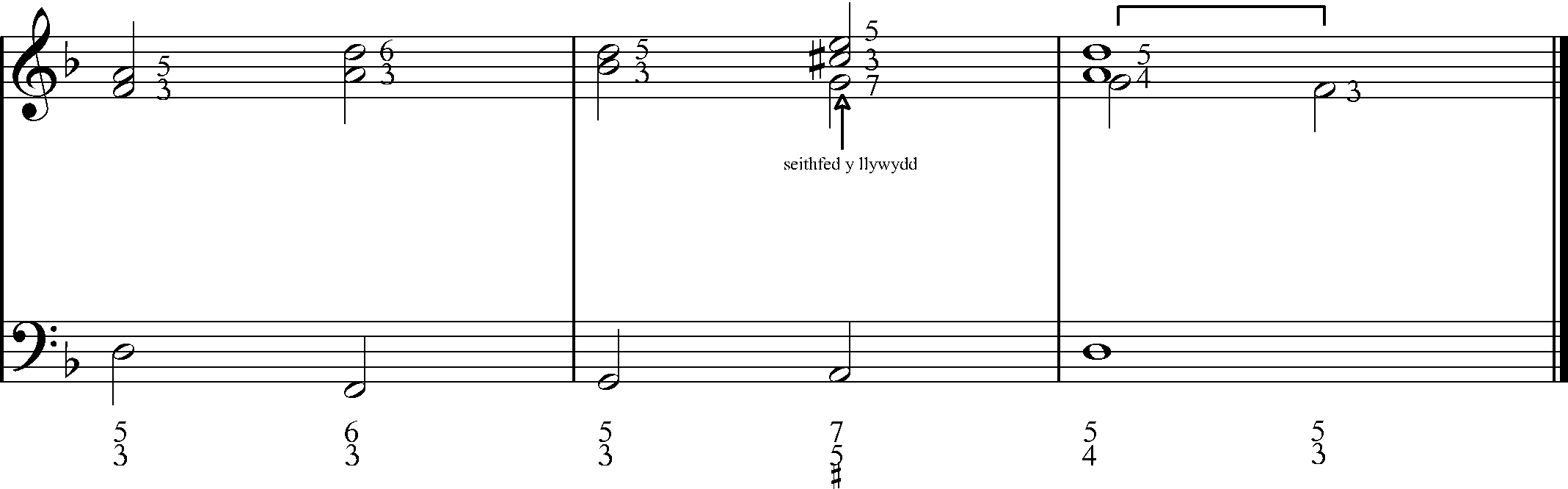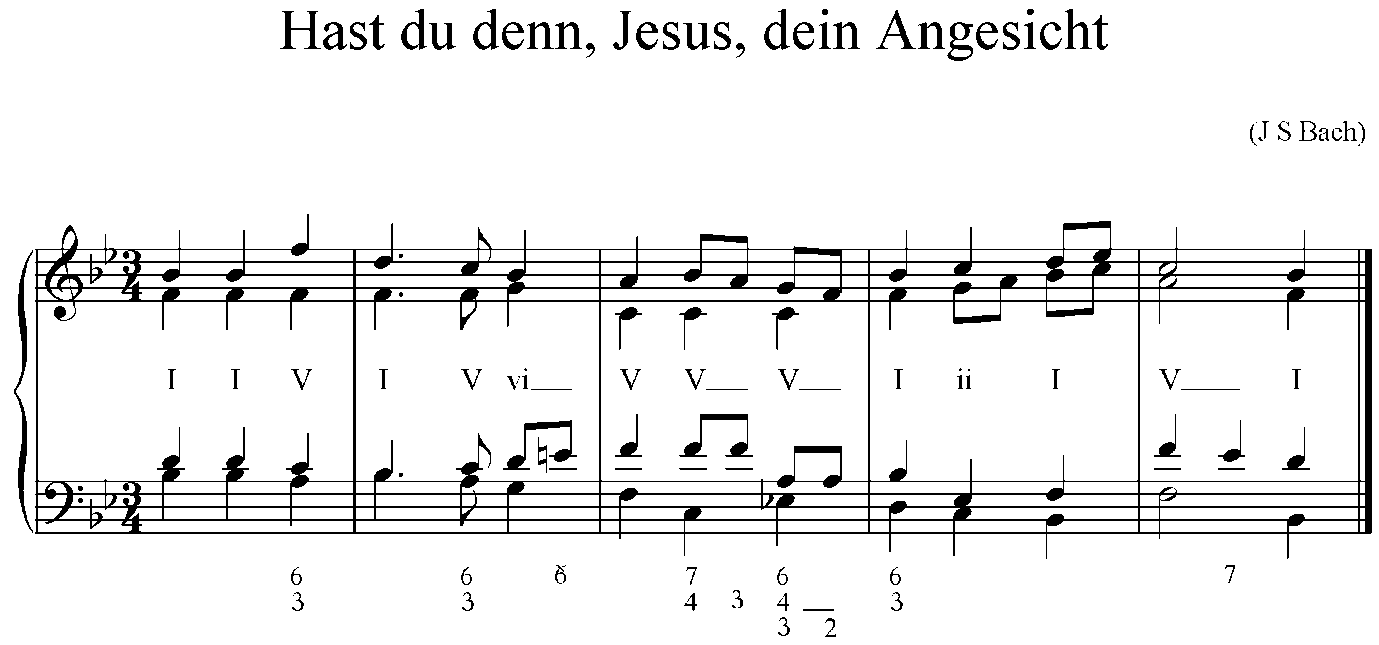# B5 | Figured Bass

• Figured bass is a short-hand method of telling the basso continuo (e.g. organ, harpsichord) what the chord is.
• Figured bass shows the notes that are in the chord above the bass note.
• The method was used extensively during the Baroque period and even as recent as some of Mozart’s works, e.g. the Requiem.

Here simply is how figured bass works:

Fig. 1

Tonic – D minor

4-3 suspensionChord i root position

Chord i first inversion

Chord iv root position

Chord V root position

Chord i root position with 4 (G) instead of 3 (F)

Chord i root position

The first chord is the tonic, which is D-F-A.

Counting from the lowest note (the bass note), F is the interval of a third above D, and A is the interval of a fifth above D. Therefore, the letter-name of the notes in the first chord are:

A = 5
F = 3
D

The second chord is :

D = 6
A = 3
F

This is a first inversion chord.

Notice that the dominant chord (V – chord A-C#-E) includes the seventh (G). The seventh can happen anywhere above the bass note, but in figured bass it is placed higher than the fifth. It also has a sharp (#). When you see an accidental (flat or sharp) alone, it refers to the third notes in the chord, that is the middle note of the basic triad (e.g. A-C#-E, D-F#-A, G-B-D).

G = 7
E = 5
C# = #
A

The quotation below is an example of a chorale harmonised by J S Bach. It was commonplace during the Baroque period to leave chords in root position with no altered notes (i.e. a common chord 8/5/3) without any figuration beneath.The symbol 6 with a line through it means that the E has been raised by half a tone, i.e. half a tone higher than the diatonic interval (it’s an E in the key-signature).

The following extract comes from the end of the first section of Mozart’s Requiem (Introitus: Requiem Aeternam). The first extract is in open score (every voice has its own line). The second is in short score format, i.e. with parts combined.

Fig. 2In this version, the composer has chosen to show the 3rd in the chords, though marking # without the figure 3 would suffice in other editions. The line shows which notes are held over into the next chord.

Fig. 3B5 Exercises